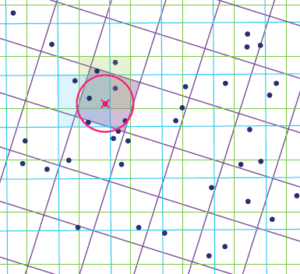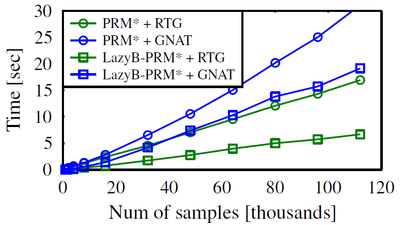# Efficient high-quality motion planning by fast all-pairs r-nearest-neighbors

## AbstractSampling-based motion-planning algorithms typically rely on nearest-neighbor (NN) queries when constructing a roadmap. Recent results suggest that in various settings NN queries may be the computational bottleneck of such algorithms. Moreover, in several asymptotically-optimal algorithms these NN queries are of a specific form: Given a set of points and a radius r report all pairs of points whose distance is at most r. This calls for an application-specific NN data structure tailored to efficiently answering this type of queries.

Randomly transformed grids (RTG) were recently proposed by Aiger, Kaplan and Sharir as a tool to answer such queries and have been shown to outperform common implementations of NN data structures in this context.

In this work we employ RTG for sampling-based motion-planning algorithms and describe an efficient implementation of the approach. We show that for motion-planning, RTG allow for faster convergence to high-quality solutions when compared with existing NN data structures. Additionally, RTG enable significantly shorter construction times for batched-PRM variants; specifically, we demonstrate a speedup by a factor of two to three for some scenarios.

Comparison to state-of-the-art nearest-neighbor libraries
To evaluate our implementation we compared our RTG implementation with the following nearest-neighbor implementations: FLANN kd-tree, ANN kd-tree, and LSH in Euclidean metric spaces (E2LSH).

For each method we measured the time for answering all-pairs r-nearest-neighbors queries for n random uniform samples from the unit d-dimensional hypercube.
The radius r = r(n) was defined as follows:We used point sets, of increasing sizes, of dimensions d = 3,6,9, and 12.

We performed the same experiment in environment cluttered with obstacles.

The following plots present our results (averaged over ten runs) for different dimensions:

Motion-planning experiments

For the following experiments we used OMPL, and compared several sampling-based motion-planning algorithms with two possible structures for  nearest-neighbors queries: (i) RTG and (ii) GNAT  (OMPL’s default).

The tested scenarios:

The roadmap construction time as a function of the number of samples for the PRM* algorithm (circle marks) on the Z-tunnel scenario in a 3D C-space (one translating robot in space) using both RTG and GNAT.

A similar experiment was performed for Lazy Batch-PRM* (marked in squares).

For these two experiments we used the Euclidean metric for distance computations.The cost as a function of time for the MPLB algorithm on the 3D Grid scenario in a 6D C-space (two translating robots in space).

The distance metric computes the sum of the distances that each robot travels (Non-Euclidean metric).

The same experiment was performed while using the Euclidean metric instead.

The success rate of finding a solution as a function of time for the MPLB algorithm on the Cubicles scenario in a 6D C-space (using both the Euclidean and the non-Euclidean metrics).

### Implementation

Our C++ implementation is available here.

The reference manual can be found here.

• Michal Kleinbort, Oren Salzman and Dan Halperin
Efficient high-quality motion planning by fast all-pairs r-nearest-neighbors
In International Conference on Robotics and Automation (ICRA), 2015 [link]

Oren Salzman
Dan Halperin
Michal Kleinbort